### Multi-Step Activities in EZStrobe – Non Identical or Non Consecutive Steps

Sometimes a modeler needs to represent an activity that consists of multiple steps as a series of shorter activities. An example is loading a flatbed with five pre-fabricated beams using a crane. This article describes how to model this situation when the time required for steps are not Independent and Identically Distributed (IID), when the number of steps required is variable, or when the multi-step activity can be interrupted between steps. The cases where none of these conditions exist can be modeled much more simply and efficiently as described in the article Multi-Step Activities in EZStrobe – Identical Consecutive Steps.

The best way to represent multi-step activities consisting of non identical continuous steps is to use an activity to model each step.

The EZStrobe network below (click it to see in detail) illustrates two such activities: One for loading one beam to a flatbed truck at the fabrication site and another for unloading it at the job site. Trucks do not explicitly circulate in the network, what circulates instead is the space required for (or occupied by) each beam in the truck. Five occupied spaces are needed for transportation to the jobsite. Five unoccupied spaces are needed for return to the fabrication site. The Load activity moves one unoccupied space waiting to load (in the BmSpotsWtLd queue) to an occupied space waiting to be transported (in the BmSpotsLdd queue) :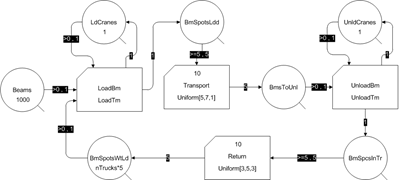The time to load (LoadTm) or unload (UnloadTm) one beam is defined using an EZStrobe Result that contains the appropriate distribution, for example Uniform[1,2] (minutes). Note that EZStrobe Results must be used in order for each activity instance to sample a new duration to obtain its time. If EZStrobe Parameters are used instead, EZStrobe will sample once at the start of the simulation to establish the value of each parameter, which will retain that value from then on. As a consequence, all instances of each activity will have the same duration rather than be sampled from the distribution to establish the time of each instance.

The network above models the exact same operation as the network in the article for identical consecutive steps. Running both networks will give the same total simulation time, the same system production in transported beams per hour, and the same utilization for the cranes that load and unload.

Since this network does not explicitly represent trucks as single units, it is not capable of providing statistics related to the waiting time and utilization of trucks.

The difference in what is represented by the several queues containing trucks compared to beam space in trucks, is illustrated by a plot of their population as a function of time. The plot below is for the first 120 minutes for trucks waiting to load when they are modeled explicitly: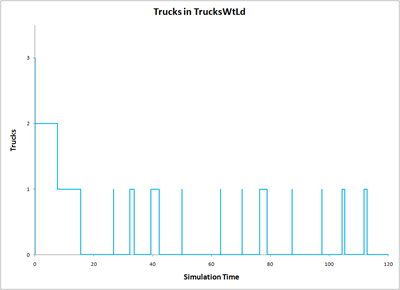Whereas the plot below is for the exact same 120 minutes for the beam space waiting to be occupied, when the truck space is modeled instead: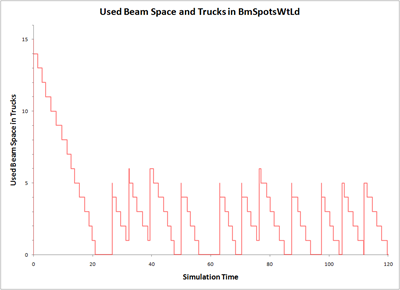The relationship between the two might be easier to appreciate in the combined chart below: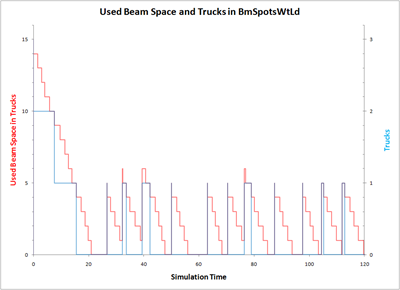As can be seen above, 4 or less beam spots mean 0 trucks,  5 to 9 beam spots mean 1 truck, 10 to 14 trucks mean 2 trucks, and so on. The number of trucks can be calculated by taking the integer part of the number of spots divided by 5, e.g., Trucks==Int[Spots/5].

Queue Integral Stats

For queues, EZStrobe allows the definition of Integral Stats, which allow the collection of statistics in terms of a larger flow unit than the one modeled in the queue. Integral Stats require a name and the number of modeled units that make up the larger unit (called the Divisor).

In the model for this article Integral Stats are defined for the BmSpotsWtLd and BmsToUnl queues. Their names are TrksWtLd and TrksWtUnld, respectively. Both Integral Stats have a divisor of 5. The screen shot below shows how to define Integral Stats for the BmSpotsWtLd  queue: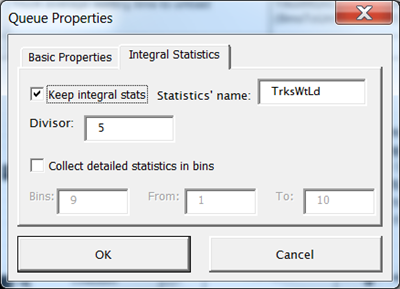When Integral Stats are defined, the simulation model report includes queue statistics from the perspective of the larger unit. These statistics are listed in the EZStrobe report under Time Weighted Collectors and includes:

• Total Time, which matches simulation time, and can be accessed in formulas using the name of the Integral Stat followed by .TtlWgt (e.g., TrksWtLd.TtlWgt).
• Current Content, which is the current content of the queue in terms of the larger unit, and can be accessed in formulas using the name of the Integral Stat (e.g., TrksWtLd).
• Average, Standard Deviation, Minimum, and Maximum Content; all in terms of the larger unit, and can be accessed in formulas using the name of the Integral Stat followed by .AveVal, .SDVal, .MinVal and .MaxVal (e.g., TrksWtLd.AveVal).

In addition, the average waiting time from the perspective of the larger unit is listed in a separate table in the EZStrobe report. Starting with EZStrobe version 3.9, this can be accessed in formulas using the name of the Integral Stat followed by _AveWait (e.g., TrksWtLd_AveWait). Note that an underscore rather than a period separates the name of the Integral Stat and AveWait. In versions of EZStrobe prior to 3.9 there is no way to access this value directly in a formula. However, it can be easily calculated in terms of other available values. For example, the average waiting time for trucks to unload can be calculated using the following formula: TrksWtLd.AveVal*TrksWtLd.TtlWgt/(BmSpotsWtLd.TotCount/5).

With the addition of Integral Stats, the model described in this article provides identical results and statistics to the model described in the article for the multi-step activities with identical consecutive steps. The model described in this article, for non-identical or non-consecutive steps, can be obtained here. It has a seed and uses multiple random number streams so that it yields the exact same output as the other model (see EZStrobe Model Options for information on seed and streams).

The Non-Identical and Consecutive Issue

The model in this page supports multi-step activities when the time for each step need not be IID, or when the steps can be interrupted. However, the actual model presented does not have either characteristic. It was presented this way so that the model is able to produce the exact same result as the model used to illustrate identical and consecutive steps. In fact, the model presented should never be used when steps are identical and continuous since the other one is cleaner and more than twice as fast in execution.

Non IID cases can be represented by setting the duration of each step with a non IID formula. An example is when the activity exhibits a learning effect, where the time of the activity is some function of 1) the number of times it has taken place, 2) the number of steps that have already taken place, or 3) any other situation that dynamically changes the parameters used to determine the time of the step.

The case of Non consecutive steps will simply include other higher priority activities that may utilize one of the resources needed for a step. For example, a crane may be needed for high priority lift that will temporarily halt the loading of beams unto a flatbed until that high priority lift is complete.

The case of a variable number steps can be modeled by appropriately replacing the “>=5,5” in the link that precedes the Transport activity, with formulas that return the appropriate number of steps required to make a whole in the current situation.

EZStrobe is based upon work supported by the National Science Foundation under Grant No. 9733267. Any opinions, findings, and conclusions or recommendations are those of the author(s) and do not necessarily reflect the views of the National Science Foundation.